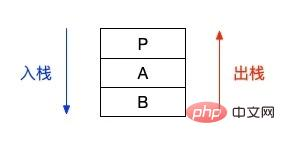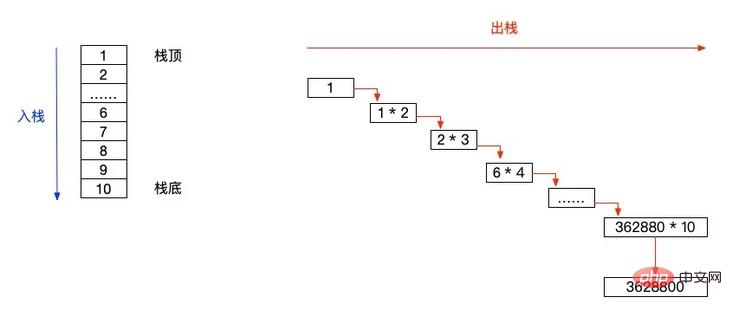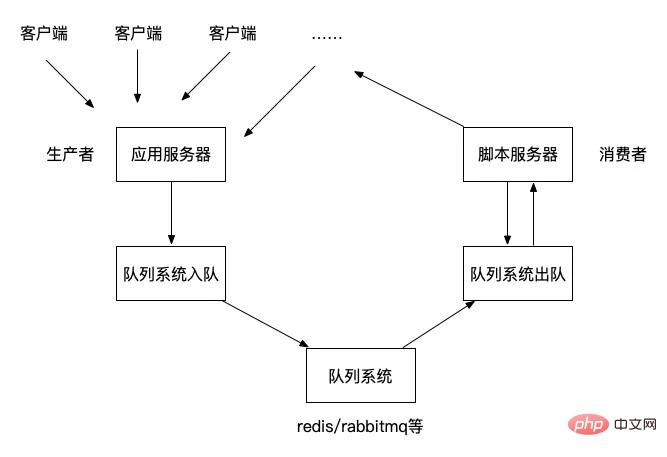# 使用栈和队列的正确姿势（附案例）

## 回文题

```\$string1 = 'abcdedcba';
\$string2 = 'abcdeedcba';
\$string3 = 'abcdefcba';

function getIsPlalindrome(\$string)
{
if (gettype(\$string) != 'string') {
return false;
}
\$strlen = strlen(\$string);
\$mid = floor(\$strlen / 2);
\$arr = [];

if (\$strlen < 2) {
return false;
}

// 入栈
for (\$i = 0; \$i < \$mid; \$i++) {
array_push(\$arr, \$string[\$i]);
}

\$j = \$mid;
\$i = \$strlen % 2 == 0 ? \$mid : \$mid + 1; // \$i 从中位数开始
for (; \$i < \$strlen; \$i++) {
\$v = \$arr[\$j - 1]; // 获得栈顶元素
if (\$v != \$string[\$i]) {
return false;
}
array_pop(\$arr); // 弹出栈顶元素
\$j--;
}
if (\$arr) {
return false;
}
return true;
}

var_dump(getIsPlalindrome(\$string1)); // bool(true)
var_dump(getIsPlalindrome(\$string2)); // bool(true)
var_dump(getIsPlalindrome(\$string3)); // bool(false)```

## 递归

```function testA()
{
echo 'A start.', PHP_EOL;
testB();
echo 'A end.', PHP_EOL;
}
function testB()
{
echo 'B start.', PHP_EOL;
echo 'B end.', PHP_EOL;
}
echo 'P start.', PHP_EOL;
testA();
echo 'P end.', PHP_EOL;

// P start.
// A start.
// B start.
// B end.
// A end.
// P end.``````function recursion(\$n)
{
if (\$n == 0 || \$n == 1) {
return 1;
}
\$result = recursion(\$n - 1) * \$n;
return \$result;
}

echo recursion(10), PHP_EOL;```## 队列应用## 总结

`https://github.com/zhangyue0503/Data-structure-and-algorithm/blob/master/3.栈和队列/source/3.3栈和队列的应用.php`• 相关标签：php
• 相关文章

相关视频